# Round Number to n Significant Figures in Excel & Google Sheets

This tutorial will demonstrate how to round a number to a specified number of significant digits in Excel & Google Sheets.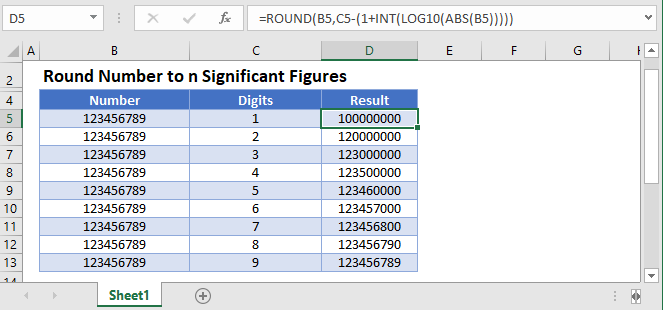## What are Significant Figures?

Significant figures are the number of digits that carry meaningful contributions to it’s measurement resolution (source: Wikipedia).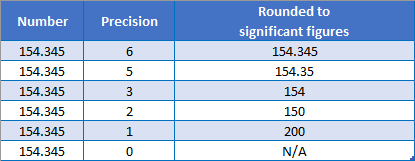## Round to n Significant Figures

This formula rounds a number to a specified number of digits (figures):

``=ROUND(A2,B2-(1+INT(LOG10(ABS(A2)))))``

This is a complicated formula. Let’s simplify it:

``=ROUND(number_to_round, sig_digits - (1 + num_digits_in_number))``

Below we will walk you through how the formula works.

### ROUND Function

The ROUND Function rounds a number to specified number of digits relative to the decimal. By using negative numbers we can round to the left of the decimal.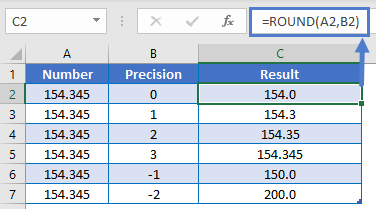As you can see, we a need a way to calculate the num_digits input in order to round to a specified number of digits. Because we know the significant digits that we want to round to, we only need a way to calculate the number of digits in a number.

### Number of Digits in Number

First let’s calculate the number of digits in the number. We will do this with the following formula:

``=INT(LOG10(ABS(A2)))``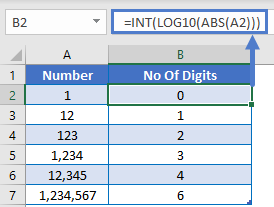Let’s walk through how the formula works…

Numbers can be stored using scientific notation:

``````1234567 = 1.234567 * 10 ^ 6
123456 = 1.23456 * 10 ^ 5
12345 = 1.2345 * 10 ^ 4
1234 = 1.234 * 10 ^ 3
123 = 1.23 * 10 ^ 2
12 = 1.2 * 10 ^ 1
``````

The exponent value in the scientific notation tells us how many digits the number contains.

### LOG10 Function

Next we can use the INT and LOG10 Functions to return the exponent from above.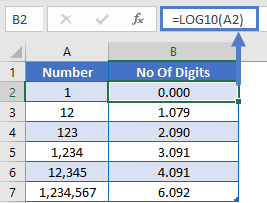How does this work? The LOG10 function returns the exponent to which 10 must be raised to produce the number.

We use the INT Function to remove the decimal value from the exponent so only the integer remains.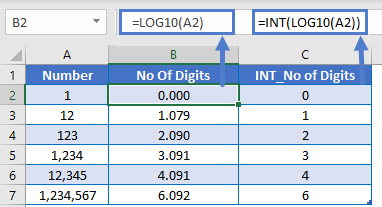### ABS Function

LOG10 will not work with negative numbers, so we also want to add in the ABS Function to calculate the absolute value, giving us the formula to calculate the number of digits in a number.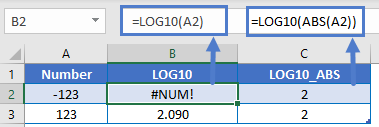### Easy Excel Automation### Number of Digits to Round

Now we know how to calculate the number of digits in a number. Remember our simplified formula above?

``=ROUND(number_to_round, sig_digits - (1 + num_digits_in_number))``

Plug in the formula to calculate the number of digits in a number:

``=ROUND(number_to_round, sig_digits - (1 + INT(LOG10(ABS(number_to_round)))))``

and let’s add cell references to arrive at our formula:

``=ROUND(A2,B2-(1+INT(LOG10(ABS(A2)))))``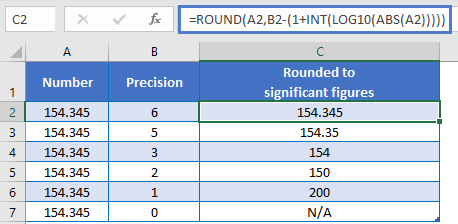## Round Number to n Significant Figures in Google Sheets

All of the above examples work exactly the same in Google Sheets as in Excel.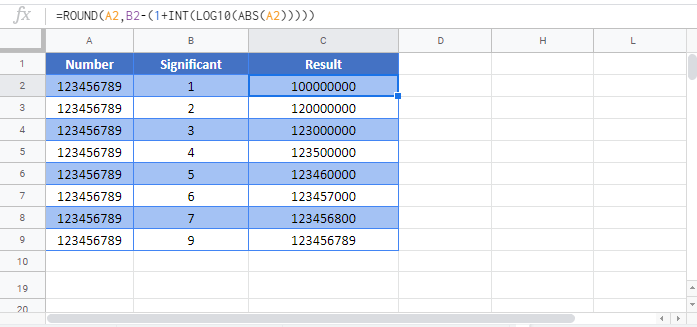### Excel Practice Worksheet

Practice Excel functions and formulas with our 100% free practice worksheets!